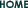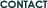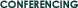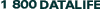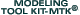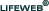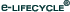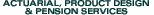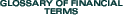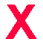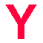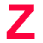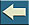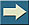IIIIIIIIIIIIIIIIIIIIIIIII
YEARLY RENEWABLE TERM (YRT)
A type of reinsurance agreement. As the name indicates, it is a one-year agreement renewable annually.
YIELD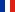A financial ratio that measures the rate of return on a capital investment. The ratio is generally expressed as a percentage of the current price. The following are some of the more common yield calculations. Definitions for these terms can be found in their normal alphabetical sequence in this listing.
YIELD TO CALL
A financial ratio that measures the rate of return on a callable bond. The yield on a bond that assumes redemption of that bond by the issuer at the first possible call date as stated in the indenture agreement.
YIELD TO MATURITY
A financial ratio that measures the rate of return on a bond's acquisition cost if the bond is held to maturity. The ratio accounts for any interest income from the bond and assumes that the bond is held and redeemed at maturity.
ZERO COUPON BONDSA bond that does not make interest payments but is sold at a deep discount. At the maturity of the bond, the full face amount of the bond is payable. The bond does result in a taxable income each year.

 IIIIIIIIIIIIIIIIIIIIIIIII